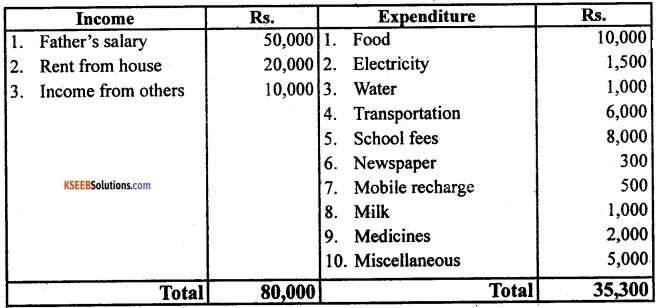# 2nd PUC Economics Model Question Paper 4 with Answers

Students can Download 2nd PUC Economics Model Question Paper 4 with Answers, Karnataka 2nd PUC Economics Model Question Papers with Answers helps you to revise the complete Karnataka State Board Syllabus and score more marks in your examinations.

## Karnataka 2nd PUC Economics Model Question Paper 4 with Answers

Time: 3 hrs 15 minutes
Max. Marks: 100

PART-A

I. Choose the correct answer (1 × 5 = 5)

Question 1.
Traditionally, the subject matter of economics has been studied under the following broad branches.
(a) Micro and macroeconomics
(b) Positive and normative
(c) Deductive and inductive
(d) None of the above
(a) Micro and macroeconomics

Question 2.
The increase in total revenue for a unit increase in the output is
(a) Marginal revenue
(b) Average revenue
(c) Total revenue
(d) Fixed revenue
(a) Marginal revenueQuestion 3.
The firms earn super normal profit as long as the price is greater than the minimum of
(a) Marginal cost
(b) Total cost
(c) Average cost
(d) Fixed cost
(c) Average cost

Question 4.
The individuals or institutions which take economic decisions are:
(a) Economic variables
(b) Economic agents
(c) Economists
(d) None of the above
(b) Economic agents

Question 5.
When demand exceeds the available output under conditions of high level of employment, this may give rise to
(a) Inflation
(b) Deflation
(c) Stabilisation
(d) None Of the above
(a) Inflation

II. Fill in the blanks (1 × 5 = 5)

Question 6.
As income increases, the demand curve for normal goods shifts towards_______
Right side.

Question 7.
For a price-taking firm marginal revenue is equal to______
Market price.

Question 8.
Savings is that part of income that is______
Not consumed.Question 9.
Non-paying users of public goods are known as
Free riders.

Question 10.
_______is a mixture of a flexible and fixed exchange rate system.
Managed floating exchange rate.

III. Match the following: (1 × 5 = 5)

Question 11.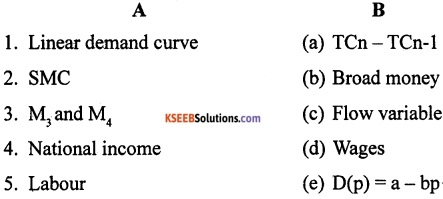1-e;2-a; 3-b; 4-c; 5-d.

IV. Answer the following questions in a sentence or a word each. (1 × 5 = 5)

Question 12.
Define marginal revenue.
Marginal revenue of a firm is defined as the increase in total revenue for a unit increase in the firm’s output. It is obtained by dividing the change in total revenue by change in quantity.

Question 13.
What is duopoly?
It is a market with two sellers or firms with many buyers. It is special case of oligopoly market.

Question 14.
What do you mean by final goods?
These are the goods which are meant for final use and will not pass through any more stages of production or transformations.Question 15.
Give the meaning of marginal propensity to save (MPS).
It is the change in savings per unit change in income. It is denoted by ‘S’ and is equal to 1-c.

Question 16.
Give the meaning of official reserve sale.
When the Reserve Bank of India sells foreign exchange when there is deficit balance of payments, it is called official reserve sale.

PART-B

V. Answer any NINE of the following in 4 sentences each. (2 × 9 = 18)

Question 17.
What is MRS?
MRS is the rate at which the consumer will substitute one product for another so that her total utility remains constant. It can be represented as follows:
MRS = ∆Y/∆X

Question 18.
What are the differences between budget line and budget set?

 Budget Line Budget Set • It is locus of different combinations of the two goods which the consumer consumes and whose price exactly equals his income. • It is also known as price line. • It is a collection of all bundles available to a Consumer at the existing price at his given level of income. • It is also known as opportunity set.

Question 19.
Give the meaning of the concepts of short run and long run.
The concepts of short-run and long run are defined aa a period simply by looking at whether all the inputs can be varied or not. It is not advisable to define short run and long run in terms of days, months or years.

In the short run, at least one of the factor – labour or capital cannot be varied and therefore, remains fixed. In order to vary the output level, the firm can vary only the other factor. The factor that remains fixed is called the fixed factor and the other factor which the firm can vary is called the variable factor.

In the long run, all factors of production can be varied. A firm in order to produce different levels of output, in the long run, may vary both the inputs simultaneously. So, in the long run there is no fixed factor.

Question 20.
State the conditions needed for profit by a firm under perfect competition.
The following conditions needed for profit by a firm under perfect competition:

• The Price P must be equal to MC.
• Marginal cost must be non-decreasing at q0.
• The firm to continue to produce, in the short run, price must be greater than the average variable cost and in the long run, price must be greater than the average cost.Question 21.
Give the meaning of shut down point.
In the short run, the shut down point is that point of minimum average variable cost where short run marginal cost curve cuts the average variable cost curve. In the long run, the shut down point is the minimum of long run average cost curve.

Question 22.
Write any two possible ways in which simultaneous shift of both demand and supply curve.
The simultaneous shifts can happen in four possible ways:

1. Both supply and demand curves shift rightwards.
2. Both supply and demand curves shift leftwards.
3. Supply curve shifts leftward and demand curve shifts rightward
4. Supply curve shifts rightward and demand curve shifts leftward.

Question 23.
Write the equation of GDPMP and GDPFC.
The equations of GDP and market prices (GDPMP), and GDP at factor cost (GDPFC) are as follows:
GDPMP = C + I + G + X-M
Where, C is consumption expenditure, I is investment expenditure; G is government’s consumption and investment expenditure; X is exports and M is imports.
GDPFC = GDPMP – NIT (where, NIT is net indirect taxes).

Question 24.
Write the difference between nominal and real GDP.

 Nominal GDP Real GDP • It is the value of GDP at current prevail­ing prices • It is evaluated at constant set of prices, i.e. by keeping base year’s price index • It is not reliable • It is reliable • It does not give real picture of economic development of a country • It gives real picture of economic development of a country

Question 25.
Differentiate the meaning of CRR and SLR.
The cash reserve ratio (CRR) is a certain percentage of bank deposits which a commercial bank is required to keep as cash reserves with itself.

The statutory liquidity ratio (SLR) refers to the ratio of deposits which the commercial banks have to maintain a certain percentage of their total deposits and time deposits with themselves in the form of liquid assets, as per the directions of RBI.

Question 26.
Distinguish between excess demand and deficient demand.
If the equilibrium level of output is more than the full employment level; it is due to the fact that the demand is more than the level of output produced at full employment level. This situation is called excess demand.

If the equilibrium level of output is less than the full employment of output, it is due to fact that demand is not enough to employ all factors of production. This situation is called deficient demand.Question 27.
Mention the non-tax revenues of the central government.
The non-tax revenues of the central government mainly consist of the following:

1. Interest receipts on account of loans by the central government.
2. Dividends and profits on investments made by the government.
3. Fees and other receipts for services rendered by the government.
4. Grants-in-aid from foreign countries and international organizations.

Question 28.
Who are free riders? Why are they called so?
If some users do not pay and it is difficult, and sometimes impossible to collect fees for the public good, such run paying users are known as free riders.

They are called so because consumers will not voluntarily pay for what they can get for free and for which there is no exclusive title (ownership) to the property being enjoyed.

Question 29.
When do surplus and deficit arise in capital account?
Surplus in capital account arises when the capital inflows are greater than capital outflows. Deficit in capital account arises when capital inflows are lesser than capital outflows.

Question 30.
Differentiate between depreciation and devaluation.
The difference between depreciation and devaluation is as follows:

 Depreciation Devaluation • Here the price of foreign currency in terms of domestic currency increases. • Here, the government deliberately makes the domestic currency cheaper by increasing exchange rate. • It happens because of market forces, i.e. demand for foreign exchange and supply of foreign exchange. • It is a deliberate action of government.

PART-C

VI. Answer any SEVEN of the following questions in 12 sentences each. (4 × 7 = 28)

Question 31.
Write a short note on a centrally planned economy.
A planned economy also called as socialistic economy is that economy where the economic activities are controlled by the central government. Here, the government takes decisions about the allocation of resources in accordance with objectives to attain economic and social welfare. Examples are Russia, China, North Korea, etc.

In centrally planned economy, the government takes decisions about the allocation of resources in accordance with the predetermined goals and objectives to attain maximum social welfare. Government decides what to produce? how to produce? and what prices are to be fixed? In a centrally planned economy, the basic economic problems are solved as follows:

1. Regarding what to produce, the government may produce those goods and services which are most useful for its society.
2. Regarding how to produce, the most suitable technique in production is adopted whether labour intensive or capital intensive in accordance with the situation in the economy.
3. Regarding whom to produce, the goods and services are produced to those people who are suffering from hunger though there is a loss.
4. It gives importance to the quality of life rather than quantity of production.
5. It focuses the resources on rapid economic development.

Question 32.
Briefly explain the budget set with the help of a diagram.
The budget set is the collection of products that the consumer can buy with is income at the prevailing market prices. The budget set is also known as opportunity set. It includes all the bundles (all possible combination of two goods) which the consumer can purchase with his given level of income.
The budget equation can be written as follows:
P1X1 + P2 X2 ≤ M.
Consider, for example, a consumer who has Rs?20 and suppose both the goods are priced at Rs.5 and are available only in integral units. The bundles that this consumer can afford to buy are (0,0), (0,1), (0,2), (0,3), (0,4), (1,0), (1,1), (1,2), (1,3), (2,0), (2,1), (2,2), (3,0), (3,1) and (4,0).

Among these bundles, (0,4), (1,3), (2,0), (2,2), (3,1) and (4,0) cost exactly Rs.20 and all the other bundles cost less than Rs.20. If both the goods are perfectly divisible, the consumer’s budget set would consist of all bundles (x1, x2) such that x1 and x2 are any numbers greater than or equal to 0 and P1X1 + P2 X2≤ M.
The budget set can be represented in a diagram as follows: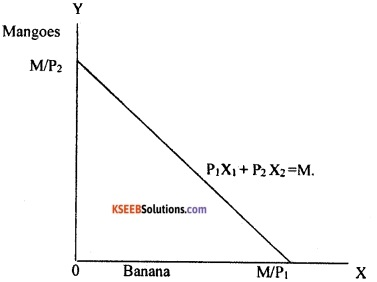Quantity of bananas is measured along the horizontal axis and quantity of mangoes is measured along the vertical axis. Any point in the diagram represents a bundle of the two goods. The budget set consists of all points on or below the straight line having the equation P1X1 + P2 X2≤ M.Question 33.
The following table gives the TP schedule of labour. Find the corresponding average product and marginal product schedules.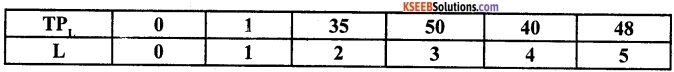Calculation of average product (AP) and marginal product (MP). AP is obtained by dividing TPL by labour (L) and MP is obtained from TPL with the help of formula TCn – TCn-1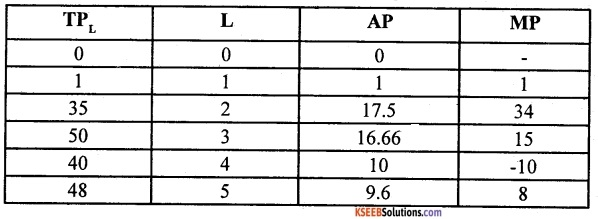Question 34.
Explain the long-run costs.
In the long run, all inputs are variable. There are no fixed costs, the total cost and the total variable cost coincide in the long run. There are two types of long-run costs. They are as follows:

1. Long-run average cost (LRAC):
The long-run average cost is the cost per unit of output produced. It is obtained by dividing the total cost by the output produced. It can be calculated as follows:
LRAC = TC/q
Where, TC is total cost and ‘q’ is quantity of output produced.

2. Long-run marginal cost:
The long-run marginal cost is the change in total cost per unit of change in output. When output changes in discrete units, then, if we increase production from q1 – 1 to q1 units of output, the marginal cost of producing q1th unit will be measured as follows:
LRMC = (TC at q1 units) – (TC at q1 – 1 units) or LRMC – TCn – TCn-1

Question 35.
Discuss the determinants of a firm’s supply curve.
A firm’s marginal cost curve is a part of its marginal cost curve. Any factor that affects a firm’s marginal cost curve is a determinant of its supply curve. Following are the two factors determining a firm’s supply curve:

a. Technological progress:
The organisational innovation by the firm leads to more production of output. That means, to produce a given level of output, the organizational innovation allows the firm to use fewer units of inputs. It is expected that this will lower the firm’s marginal cost at any level of output, i.e. there is a rightward shift of the MC curve. As the firm’s supply curve is essentially a segment of the MC curve, technological progress shifts the supply curve of the firm to the right. At any given market price, the firm now supplies more quantity of output.

b. Input prices:
A change in the prices of factors of production (inputs) also influences a firm’s supply curve. If the price of input (e.g. wage) increases, the cost of production also increases. The consequent increase in the firm’s average cost at any level of output is usually accompanied by an increase in the firm’s marginal cost at any level of output which leads to upward shift of the MC curve. That means, the firm’s supply curve shifts to the left and the firm produces less quantity of output.

Question 36.
Explain the features of perfect competition.
Perfect competition is a market where there will be existence of large number of buyers and sellers dealing with homogenous products. It is a market with highest level competition.

1. Large number of sellers and buyers:
The first condition which a perfectly competitive market must satisfy is concerned with the sellers’ side of the market. The market must have such a large number of sellers that no one seller is able to dominate in the market. No single firm can influence the price of the commodity. The sellers will be the firms producing the product for sale in the market. These firms must be all relatively small as compared to the market as a whole.

Their individual outputs should be just a fraction of the total output in the market. There must be such a large number of buyers that no one buyer is able to influence the market price in any way. Each buyer should purchase just a fraction of the market supplies. Further, the buyers should have any kind of union or association so that they compete for the market demand on an individual basis.

2. Homogeneous products:
Another prerequisite of perfect competition is that all the firms or sellers must sell completely identical or homogeneous goods. Their products must be considered to be identical by all the buyers in the market. There should not be any differentiation of products by sellers by way of quality, colour, design, packing or other selling conditions of the product.

3. Free entry and free exit for firms:
Under perfect competition, there is absolutely no restriction on entry of new firms in the industry or the exit of the firms from the industry which want-to leave. This condition must be satisfied especially for long period equilibrium of the industry.

If these three conditions are satisfied, the market is said to be purely competitive. In other words, a market characterized by the presence of these four features is called purely competitive. For a market to be perfect, some conditions of perfection of the market must also be fulfilled.

4. Price taker:
The single distinguishing character of perfect competition is the price-taking behaviour of the firms. A price-taking firm believes that if it sets a price above the market price, it will be unable to sell any quantity of the good that it produces. On the other hand, if the firm set the price less than or equal to the market price, the firm can sell as many units of the good as it wants to sell.

The firms in the perfect competitive market are price takers. That means, the producers will continue to sell their goods and services in the price existing in the market. Firms have no control over the price of the product.

5. Information is perfect:
Price taking is often thought to be a reasonable assumption when the market, has many firms and buyers have perfect information about the price prevailing in the market. Since all firms produce the same good and all buyers are aware of the market price, the firm in question loses all its buyers if it raises price.

Question 37.
Briefly explain in what way macroeconomics is different from microeconomics.
The micro and macroeconomics are distinguished on the following grounds:
1. Scope:

• Microeconomics study in individual units so its scope is narrow.
• Macroeconomics study in aggregates, so its scope is wider.

2. Method of study:

• The microeconomics follows slicing method as it studies individual unit.
• The macroeconomics follows lumping method as it studies in aggregates.

3. Economic agents:

• In microeconomics, each individual economic agent thinks about its own interest and welfare.
• In macroeconomics, economic agents are different among individual economic agents and their goal is to get maximum welfare of a country.

4. Equilibrium:

• Microeconomics studies the partial equilibrium in the country.
• Macroeconomics studies the general equilibrium in the economy.

5. Domain:

• Microeconomics consists of theories like consumer’s behaviour, production, and cost, rent, wages, interest, etc.
• Macroeconomics comprises of theory of income, output, and employment, consumption function, investment function, inflation, etc.Question 38.
Explain the examples of planned accumulation and decumulation of inventories.
Inventories are the unsold goods, unused raw materials or semi-finished goods which a firm carries from a year to the next year. Change in inventories may be planned or unplanned. A planned change in inventories is the change in the stock of inventories which has occurred in a planned way. The planned accumulation and decumulation of inventories are explained with example as follows.

Suppose a firm wants to increase the inventories from 200 T-shirts to 400 T-shirts during the year. Expecting sales of 2000 T-shirts during the year, the firm produces 2000 + 200 = 2200 T-shirts. If the sales are actually 2000 T-shirts, the firm ends up with a rise of inventories. The new stock of inventories is 400 T-shirts, which was planned by the firm. This is planned accumulation of inventories.

On the other hand, if the firm had wanted to reduce the inventories from 200 to 50, then it would produce 2000 – 150 = 1850 T-shirts. This is because it plans to sell 150 T-shirts out of the inventory of 200 T-shirts it started with. Then the inventory at the end of the year becomes 200 – 150 = 50 T-shirts, which the firm wants. If the sales turn out to be 2000 T-shirts as expected by the firm, the firm will be left with the planned reduced inventory (decumulation) of 50 T-shirts.

These are the two instances of planned accumulation and planned decumulation of inventories.

Question 39.
Give the meaning of transaction motive and speculative motive of demand for money and liquidity trap.
1. Transaction motive:
Transaction motive demand for money refers to holding money to carry out transactions. If we receive our income weekly and make payments on the first day of every week, we need not hold any cash balance throughout the rest of the week. But our expenditure patterns do not normally match our receipts. People earn incomes at discrete points in time and spend it continuously throughout the interval.
The transaction demand for money is represented as follows:
MdT = k. T
Where, T is the total value of transactions in the economy over unit period and k is a position fraction.

2. Speculative motive:
Some people hold cash to invest bn shares, debentures, gold, immovable properties, etc. The speculative demand for money refers to the demand for money that people hold as idle cash to speculate with the aim of earning capital gains and profits. The speculative demand for money can be written as follows:
$$M_{s}^{d}=\frac{r_{\max }-r}{r-r_{\min }}$$
Where, r is the market rate of interest and rmax and rmin are the upper and lower limits of r, both positive constants. It clearly states that as r decreases from rmax to rmin, the value of speculative demand for money decreases from zero to infinity.

Question 40.
Briefly explain the balance of trade.
Balance of trade is the difference between the value of exports and value of imports of goods of a country in a given period of time. Export of goods is entered as a credit item in balance of trade. Import of goods is entered as a debit item in balance of trade. It is also called as trade balance.

Balance of trade is said to be in balance when exports of goods are equal to the imports of goods, i.e. balanced balance of trade.
Surplus balance of trade arises if country’s exports of goods are more than its imports.

Deficit balance of trade arises if a country’s imports of goods are more than its exports. Balance of trade is narrow concept and it may not show the international economic position of an economy. It gives partial picture of international transactions and it is less reliable. It does not include net invisibles, i.e. the difference between the value of exports and value of imports of invisibles (services) of a country in a given period of time.

Question 41.
Write the chart of components of currentaccount.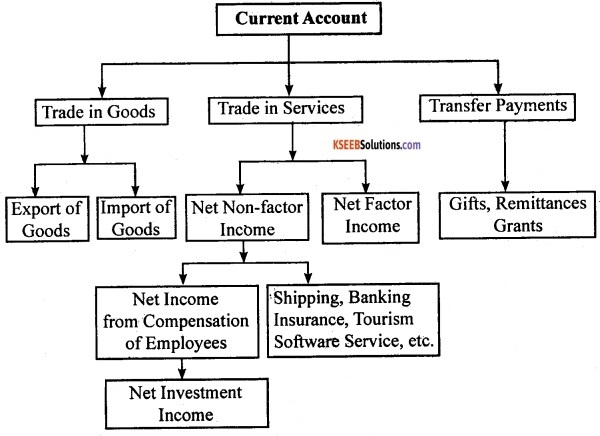PART-D

VII. Answer any Six of the following questions in 20 sentences each. (6 × 4 = 24)

Question 42.
Explain the optimal choice of consumer with the help of diagram.
It is assumed that the consumer chooses her consumption bundle on the basis of her taste and preferences over the bundles in the budget set. It is generally assumed that the consumer has well defined preferences over the set of all possible bundles. She can compare any two bundles. In other words, between any two bundles, she either prefers one to the other or she is indifferent between the two goods.

It is further assumed that the consumer is a rational individual. A rational individual clearly knows’ what is good or what is bad for her and in any given situation, she always tries to achieve the best for herself. From the bundles which are available to her, a rational consumer always chooses the one which gives her maximum satisfaction. The consumer always tries to move to a point on the highest possible indifference curve given her budget set.

Thus, the optimum point would be located on the budget line. A point below the budget line cannot be the optimum. Compared to a point below the budget line, there is always some point on the budget line which contains more of at least one of the goods and no less of the other. Thus, the consumer’s preferences are monotonic.

The point at which the budget line is tangent to one of the indifference curves would be the optimum i choice of consumer. This is because, the budget line other than the point at which it touches the indifference curves lies on a lower indifference curve is considered as inferior. So such a point cannot be the consumer’s optimum. The optimum bundle is located on the budget line at the point where the budget line is tangent to an indifference curve.
This can be explained with the help of the following diagram: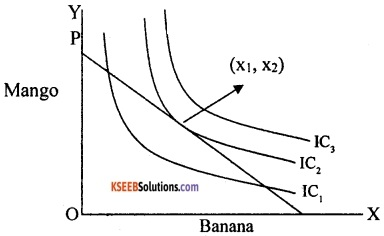In the above diagram, PQ is budget line, IC1, IC2and IC3 are indifference curves showing different levels of satisfaction. Banana is measured in OX axis and mango is measured in OY axis.

The above diagram illustrates the consumer’s optimal choice also known as consumer’s equilibrium. At (x1, x2), the budget line PQ is tangent to the indifference curve IC2. The indifference curve just touching the budget line is the highest possible indifference curve given the consumer’s budget set.

Bundles, on the indifference curve above IC2 are not affordable. Points on the indifference curve IC2 are certainly inferior to the points on the IC2 as they lie on IC1. Therefore, (x1, x2) is the consumer’s optimum bundle.Question 43.
Explain the short run supply curve of a firm with the help of a diagram.
Supply of a firm refers to the quantity that it chooses to sell at a given price, given technology and given prices of factors of production. Supply curve of a firm shows the levels of output that the firm chooses to produce corresponding to different values of the market price by keeping technology and prices of factors of production constant.

1. Short-run supply curve of a firm:
Let us derive a firm’s short-run supply curve. The derivation of the & supply curve can be split into two parts viz., firm’s profit-maximizing output level when the market price is greater than or equal to minimum average variable cost and the firm’s profit-maximizing output level when the market price is less than the minimum average variable cost.
Case 1:
Price or average revenue greater than or equal to the minimum AVC:
This can be explained with the help of the following diagram.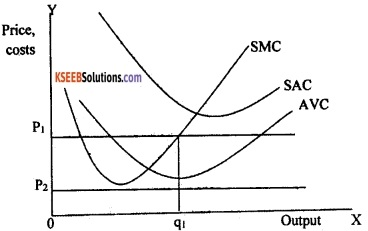If the market price is P1, which exceeds the minimum of AVC, the firm starts out by equating P1 with SMC on the rising part of the SMC curve which leads to the output level q1 But the AVC at q1 does not exceed the market price P1. Thus, when the market price is P1, the firm’s output level in the short run is equal to q1.

Case 2:
Price is less than minimum AVC: If the market price is P2 which is less than the minimum AVC, at all positive output levels, AVC exceeds P2 In other words, it cannot be the case that the firm supplies a positive output. So, if the market price is P2 the firm produces zero output.

Combining both the cases, we can conclude that a firm’s short-run supply curve is the rising part of the short-run marginal curve from and above the minimum average variable cost together with zero output for all prices strictly less than the minimum AVC. This can be represented in the following diagram: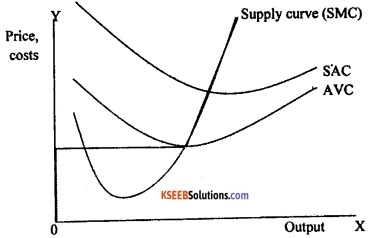In the above diagram, the short-run supply curve of a firm, which is based on its short-run marginal cost curve and average variable cost is represented by the curve which rises from the minimum point of AVC curve. The bold line represents the short-run supply curve.

Question 44.
Explain the short-run equilibrium of a monopoly firm with the help of the simple case of zero cost.
Every monopolist aims at maximizing profit. Here, we try to analyze the profit-maximizing behaviour to determine the quantity produced by a monopoly firm and price at which it is sold.

Let us imagine that there exists a village situated far way from other villages. In this village, there is exactly one well from which water is available. All residents are completely dependent for their water requirements on this well. The well is owned by one person who is able to prevent others from drawing water from it except through purchase of water.

The person who purchases the water has to draw the water out of the well. The well owner is thus a monopolist firm which bears zero cost in producing the good. We shall analyse this simple case of a monopolist bearing zero costs to determine the amount of water sold and the price at which it is sold.

The short ran equilibrium of the monopolist with zero cost can be explained with the help of the following diagram: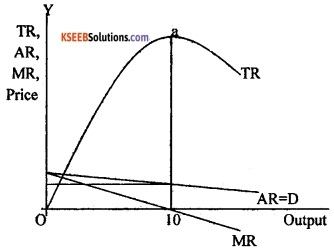In the above diagram, TR, AR and MR curves are revenue curves. The profit received by the firm equal the revenue received by the firm minus the cost incurred. Since TC is zero, profit is maximum when TR is maximum. This occurs when output is of 10 units. This is the level when.MR equals zero. The amount of profit is given the length of the vertical line segment from ‘a’ to the horizontal axis.

Question 45.
Briefly explain the expenditure method of measuring GDP.
Expenditure method is the alternative way to calculate the GDP by looking at the demand side of the products. Here the aggregate value of the output in the economy by expenditure method will be calculated in the following way.

In this method, we add the final expenditures that each firm makes. Final expenditure is that part of expenditure which is undertaken not for intermediate purposes. If the baker buys Rs.50 worth of wheat from the farmers is considered as intermediate good and the final expenditure received by the baker is 200. Then the aggregate value of output of the economy is Rs.200 + Rs.50 = Rs.250.

Let us assume that firm i makes the final expenditure .on the following accounts:

1. Final consumption expenditures on the goods and services by households denoted as Ci
2. Final investment expenditure incurred by the firms on capital goods denoted as Ii
3. The expenditure that the government makes on the final goods and services produced by the firm, denoted as Gi
4. The export revenues that firm i earns by selling its goods and services abroad denoted as Xi

Now the total final consumption, investment, government and export expenditures received by the firm i. Now GDP according to the expenditure method is expressed as follows:
GDP = $$\sum_{i=1}^{N}$$ RVi = C + I + G + X- M
$$\sum_{i=1}^{N}$$RVf is the sum of final consumption expenditure-C, investment -I, government – G and exports -X and imports-M, received by all the firms in the economy.Question 46.
Requirement of reserves acts as a limit to money (credit) creation. Explain.
The RBI decides a certain percentage of deposits which every bank must keep as reserves. This is done to ensure that no bank is over lending. This is a legal requirement and is binding on the banks. This is called the CRR (cash reserve ratio).

Apart from the CRR, banks are also required to keep some reserves in liquid form in the short term. This ratio is called SLR (Statutory Liquidity Ratio). The statutory requirement of the reserve ratio acts as a limit to the amount of credit that banks can create.

For example, let us assume that Canara Bank starts with a deposit of Rs.1000 made by Mr.X. The U . reserve ratio is 20%. Thus, Canara Bank has Rs.800 (1000 – 200=800, i.e. 20% of 1000 is deducted) to lend and the bank lends out of Rs.800 to Mr.Y, which is shown in the bank’s deposits in the next round as liabilities, making a total of Rs. 1800 as deposits. Now Canara bank is required to keep 20% of 1800, i.e, 360 as cash reserves.

The bank had started with Rs.1000 as cash. Since it is required to keep only Rs.360 as reserves it lends Rs.640 (1000-360=640)-. The bank lends out Rs.640 to Mr. Z. This in turn shows up in the bank, as deposits. The process keeps repeating itself till all the required reserves become Rs. 1000. The required reserves will be Rs. 1000 only when the total deposits become Rs.5000. This is because, for deposits of Rs.5000, cash reserves would have to be Rs.1000 (20% of 5000= 1000).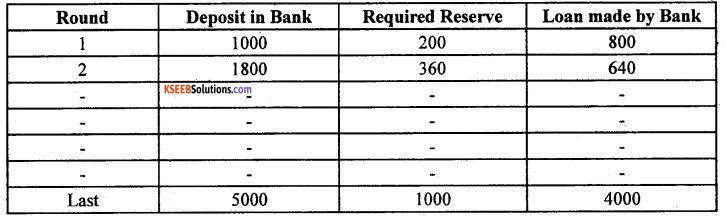In the above table, t le first column lists each round. The second column depicts the total deposits with the bank at the beginning of each round. 20% of these deposits need to be deposited with the RBI as required reserves (3rd column). What the bank lends in each round gets added to the deposits with the bank in the 2nd round 4th column indicates the loans made by the banks.

Question 47.
Explain the changes in taxes with the help of a diagram.
We know that a decrease in tax leads to increase in disposable income at each level of income. This. shifts the aggregate expenditure schedule upwards by a fraction c of the decrease in taxes. This can be shown in the following diagram: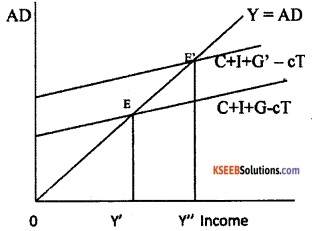In the above diagram, income is measured in X-axis and aggregate demand is measured in Y-axis. When the tax is decreased, there will be increase in consumption and output and the tax multiplier is negative. The tax multiplier is given as:
∆Y/∆T = -c/1-c.
It shows that the tax multiplier is smaller in absolute value. This is because an increase in government spending directly affects total spending whereas taxes enter the multiplier process through their impact on disposable income, which influences household consumption. Thus, with decrease in taxes, consumption and total spending increases in the first instance by c∆T.

For example, assume that the marginal propensity to consume is 0.8. The government expenditure multiplier will then be.
1/1-c = 1/1 – 0.8 = 1/0.2 = 5.

For an increase in government spending by 100, the equilibrium income will increase by 500 (5 × 100). The tax multiplier is given by -c/1-c = -0.8/1—0.8 = -0.8/0.2 = -4. Therefore, a tax cut of 100 will increase equilibrium income by 400.

If the different values of the marginal propensity to consume are taken to calculate the values of the two multipliers, we find that the tax multiplier is always one less in absolute value than the government expenditure multiplier. If an increase in government spending is matched by an equal increase in taxes, so that the budget remains balanced, output will rise by the amount of the increase in government spending.

Question 48.
Explain the foreign exchange market with fixed exchange rates with the help of a diagram.
Under fixed exchange rate system, the government decides the exchange rate at a particular level. The foreign exchange market with fixed exchange rates can be explained with the help of following diagram: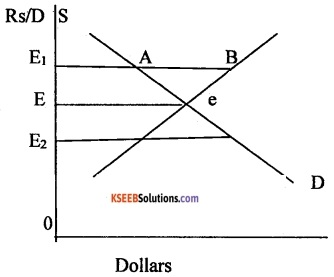In the above diagram, the market-determined exchange rate is e where demand and supply intersect. However, if the government wants to encourage exports for which it needs to make rupee cheaper for foreigners it would do so by fixing a higher exchange rate say, Rs.70 per dollar from the current exchange rate of Rs.65 per dollar.

Thus, the new exchange rate set by the government is E, where E, is greater than E. At this exchange rate, supply of dollars exceeds the demand for dollars. The RBI intervenes to purchase the dollars for rupees in the foreign exchange market in order to absorb this excess supply which has been marked as AB in the diagram.

Thus, by interfering, the government can maintain any exchange rate in the economy. If the government wants to set an exchange rate at a level E2 there would be an excess demand for dollars, the government would have to withdraw dollars from its past holds of dollar. If the government fails to do so, it will encourage black market transactions.

PART-E

VIII. Answer any two of the following project-oriented questions. (5 × 2=10)

Question 49.
Find the missing products of the following table.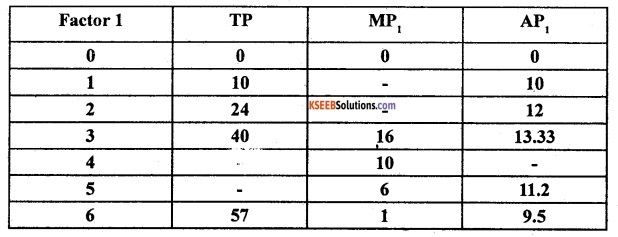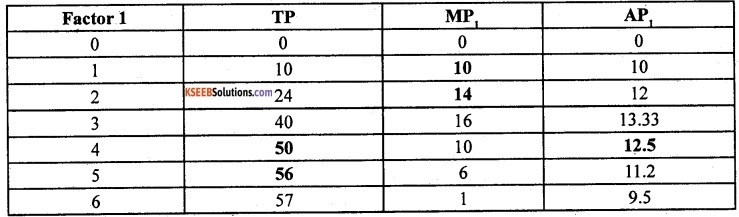Question 50.
Write a note on demonetisation.
Demonetisation was a new step taken by the Government of India on 8th November 2016. It was introduced to tackle the problem of corruption, black money, terrorism and Circulation of fake currency in the economy. Old currency notes of Rs.500 and Rs. 1000 were no longer legal tender. New currency notes in denomination of Rs.500 and Rs.2000 were introduced. The public were advised to deposit old currency notes in their bank account till 31st of March 2016 without any declaration and up to 31st March 2017 with the RBI with declaration.

In order to avoid a complete breakdown and scarcity of cash, government allowed exchange of Rs.4000 old currency notes with new currency restricting to a person per day. Further, till 12th December 2016, old currency notes were acceptable as legal tender at petrol pumps, government hospitals and for payment of government dues like taxes, power bills, etc.

This initiative had both appreciation and criticism. There were long queues outside banks and ATM centres. There was acute shortage of currency notes and had adverse effect on economic activities. But now, normalcy has returned.

The demonetisation also has positive effects. It improved tax compliance as a large number of people were bought in the tax ambit. The savings of individual were channelized into the formal financial system. As a result, banks have more resources at their disposal which can be used to provide more loans at low rate of interest.

Demonetisation helps in curbing black money, reducing tax evasion and corruption will decrease. It also helps in tax administration in another way, by shifting transaction out of the cash economy into the formal payment system. Now a days, households and firms have started to shift from cash payment to electronic payments.Question 51.
Prepare a budget on monthly income and expenditure of your family.